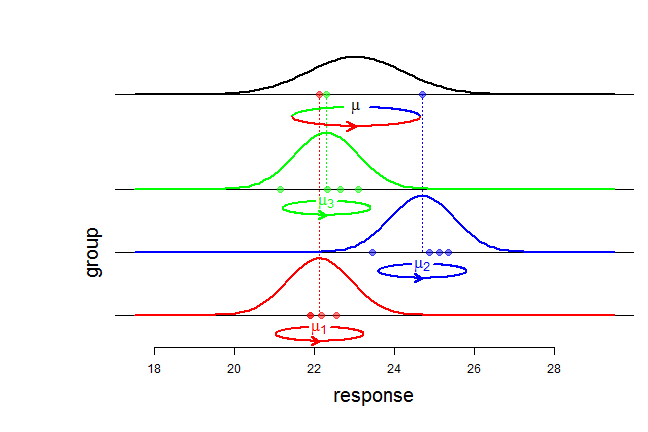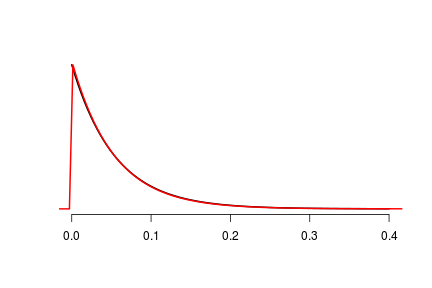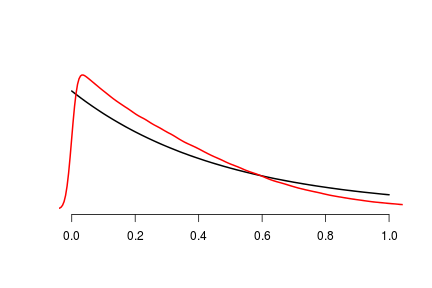1. Inference in the balanced one-way random effect ANOVA

2015-03-12
Source

The one-way random effect ANOVA model is the simplest linear Gaussian model with random effects: one random factor, and no other factor or covariate. However, methods of inference to get estimates, confidence intervals for various parameters (variance components, coefficient of variation), prediction intervals and tolerance intervals, are not so well-known. This is what we provide in this article, in the simplest situation : the case of balanced data (at the end we give a possible way to handle the unbalanced situation).

Recall that, denoting by $y_{ij}$ the $j$-th response in group $i$. this model assumes that the responses $y_{ij}$ are generated in two steps. First, the group means $\mu_i$ are independently generated according to a Gaussian distribution ${\cal N}(\mu, \sigma^2_b)$ where $\mu$ is the overall mean and $\sigma^2_b$ is the so-called between variance. Second, the responses $y_{ij}$, $j =1,\ldots,J$, for each group $i$, are independently distributed according to a Gaussian distribution ${\cal N}(\mu_i, \sigma^2_w)$ with within variance $\sigma^2_w$ and mean $\mu_i$. Shortly, the model can be written: $\begin{cases} \mu_i \sim_{\text{iid}} {\cal N}(\mu, \sigma^2_b) & i=1,\ldots,I \\ (y_{ij} \mid \mu_i) \sim_{\text{iid}} {\cal N}(\mu_i, \sigma^2_w) & j=1,\ldots,J \end{cases}.$If you like the moment of inertia representation of the variance on this picture (I do), see my article about it.

Throughout the article, we will use the following function to get the within sum-of-squares $SS_w$ and the between sum-of-squares $SS_b$:

SOS <- function(y, group){
require(dplyr)
return(data.frame(y=y,group=group) %>% mutate(mean=mean(y)) %>% group_by(group) %>% mutate(groupmean=mean(y)) %>% ungroup %>% do(summarise(., SSw=crossprod(y-groupmean), SSb=crossprod(groupmean-mean))) %>% as.list)
}

Bayesian posterior distributions

All Bayesian methods we provide are based on the posterior distributions generated by this code. I???m using a data.table for storing the stimulations.

ranova <- function(y, group, nsims=50000){
require(data.table)
group <- factor(group)
I <- length(levels(group))
if(!all(I*as.numeric(table(group))==length(y))){ stop("balanced only!") }
J <- length(y)/I
SS <- SOS(y, group) #  sum of squares SSb SSw
SSb <- SS$SSb; SSw <- SS$SSw
omean <- mean(y)
k <- tot <- 0
z <- rnorm(nsims)
ETAb <- ETAw <- rep(NA,nsims)
while(k < nsims){
tot <- tot +1
etab <- SSb/rchisq(1, I-1)
etaw <- SSw/rchisq(1, I*(J-1))
if(etab>etaw){
k <- k+1
ETAb[k] <- etab
ETAw[k] <- etaw
}
}
out <- data.table(mu=omean + sqrt(ETAb/I/J)*z, sigma2w=ETAw, sigma2b=(ETAb-ETAw)/J)
reject <- 100*(tot-nsims)/tot
cat("Rejection percentage: ", round(reject,1), "%")
setattr(out, "reject", reject)
return(out)
}

These posterior distributions can be found in Krishnamoorthy & Mathew???s book. I believe they are derived in Box & Tiao???s book.

As we can see in the body of the function, the algorithm firstly generates simulations of a couple $(\eta_w, \eta_b)$ whose joint distribution is given two inverse-Gamma distributions ${\cal IG}\left(\frac{I(J-1)}{2},\frac{SS_w}{2}\right)$ and ${\cal IG}\left(\frac{I-1}{2},\frac{SS_b}{2}\right)$ conditionned to $\eta_b > \eta_w$, and then $\sigma^2_w=\eta_w$ $\sigma^2_b=\frac{\eta_b-\eta_w}{J}$. The ranova function also prints the percentage of rejected simulations (those for which etab<etaw) and also returns it as an attribute of the data.table containing the simulations.

Note that $\eta_b = J\tau^2$ where $\tau^2=\sigma_b^2+\frac{\sigma_w^2}{J}$ is the variance of a group mean $\bar y_{i\bullet}$. Given the sum of squares $SS_w$ and $SS_b$, the probability of rejection is, using abusive notation, \begin{align} R(SS_w,SS_b) & = \Pr\left(\frac{\chi^2_{I(J-1)}}{\chi^2_{I-1}} < \frac{SS_w}{SS_b}\right) \\ & = \Pr\left({\cal Beta}'\left(\frac{I(J-1)}{2}, \frac{I-1}{2}\right) < \frac{SS_w}{ SS_b} \right) \\ & = \Pr\left({\cal Beta}\left(\frac{I(J-1)}{2}, \frac{I-1}{2}\right) < \frac{SS_w}{SS_w + SS_b} \right). \end{align}

Moreover, as is well known or as shown here, the sum of squares $SS_w$ and $SS_b$ are independent and $SS_w \sim \sigma^2_w\chi^2_{I(J-1)}$ and $SS_b \sim J\tau^2\chi^2_{I-1}$.

Therefore the rejection probability over repeated sampling is $R = \Pr\left(\frac{{\cal Beta}'\left(\frac{I(J-1)}{2}, \frac{I-1}{2}\right)}{{\cal Beta}'\left(\frac{I(J-1)}{2}, \frac{I-1}{2}\right)} < \frac{\sigma^2_w}{J\tau^2} \right).$ Let $\rho:=\dfrac{\sigma^2_w}{\sigma^2_w+\sigma^2_b}$ be the ratio of the within-variance over the total variance, and note that $\dfrac{\sigma^2_w}{J\tau^2} = \dfrac{\rho}{\rho+J(1-\rho)}$ (an increasing function of $\rho$). There is no elementary cumulative distribution fonction for the ratio of the two independent Beta prime distributions, but we can easily plot $R$ in function of $\rho$ with the help of simulations. We use Javascript in order to include interactivity for the choice of $I$ and $J$, and the Javascript flot library to render the plot:

As an exercise, you can check why the probability of rejection always is $50\%$ for $\rho=1$.

Simulated data

Throughout the article, we will illustrate the methods on two datasets, dat1 and dat2. I load the magrittr package to use the pipe operator %>%.

library(magrittr)
set.seed(314)
I <- 3
J <- 6
mu <- 10
sigmab <- 2
sigmaw <- 1
dat1 <- data.frame(sapply(rnorm(I,mu,sigmab), function(mu) rnorm(J,mu,sigmaw))) %>%
setNames(LETTERS[1:I]) %>% stack %>% setNames(c("y", "group"))
sigmab <- 1
dat2 <- data.frame(sapply(rnorm(I,mu,sigmab), function(mu) rnorm(J,mu,sigmaw))) %>%
setNames(LETTERS[1:I]) %>% stack %>% setNames(c("y", "group"))
# stacked datasets
dat <- rbind(cbind(data="dat1", dat1), cbind(data="dat2", dat2))

The between-variance $\sigma_b^2$ is the only difference between the parameters used to generate our two datasets ($4$ vs $1$). We can already store the posterior simulations of the Bayesian method:

sims1 <- with(dat1, ranova(y, group, nsims=1e6))
## Rejection percentage:  0 %
sims2 <- with(dat2, ranova(y, group, nsims=1e6))
## Rejection percentage:  19.2 %
# stacked simulations in a data.table
sims <- rbind(sims1[, "data":="data1"], sims2[,"data":="data2"])
setkey(sims, data)

Note that the theoretical rejection percentages could be calculated with our above formula of $R(SS_w,SS_b)$:

by(dat, dat$data, FUN=function(data){ with(data, with(SOS(y,group), round(100*pbeta(SSw/(SSw+SSb), I*(J-1)/2, (I-1)/2), 1) ) ) }) ## dat$data: dat1
##  0
## --------------------------------------------------------
## dat$data: dat2 ##  19.1 Because there is no rejection for the first dataset, the posterior distribution of $\eta_b$ is ${\cal IG}\left(\frac{I-1}{2},\frac{SS_b}{2}\right)$, as confirmed by the density plot below (see this stats.stackexchange discussion if you wonder why I use the logspline package): with(dat1, { ssb <- J*crossprod(aggregate(y~group, FUN=mean)$y-mean(y))
curve(dgamma(x,(I-1)/2, ssb/2), to=0.4, lwd=2, xlab=NA, ylab=NA, axes=FALSE)
})
axis(1)
library(logspline)
x <- 1/with(sims1[sample(nrow(sims1),1e4)], J*sigma2b+sigma2w)
plot(logspline(x), add = TRUE, col = "red", lwd = 2)But not for the second dataset:

with(dat2,
{
ssb <- J*crossprod(aggregate(y~group, FUN=mean)$y-mean(y)) curve(dgamma(x,(I-1)/2, ssb/2), to=1, ylim=c(0,2.6), lwd=2, xlab=NA, ylab=NA, axes=FALSE) }) axis(1) lines(density(1/with(sims2, J*sigma2b+sigma2w)), col="red", lwd=2)Confidence interval about the overall mean Frequentist method There???s a straightforward way to get a confidence interval about the overall mean $\mu$: just take the group means and draw a usual Gaussian confidence interval about their mean: by(dat, dat$data, function(data){
aggregate(y~group, data=data, FUN=mean) %>% lm(y~1, .) %>% confint
})
## dat$data: dat1 ## 2.5 % 97.5 % ## (Intercept) 4.304683 13.03132 ## -------------------------------------------------------- ## dat$data: dat2
##                2.5 %   97.5 %
## (Intercept) 8.419673 11.37769

Bayesian method

Throughout the article we always use equi-tailed credible intervals.

quantile(sims["data1"]$mu, c(2.5,97.5)/100) ## 2.5% 97.5% ## 4.29467 13.03949 quantile(sims["data2"]$mu, c(2.5,97.5)/100)
##      2.5%     97.5%
##  8.242078 11.563506

For the first dataset, the Bayesian interval is close to the frequentist interval. We can see why: when $R(SS_w,SS_b)=0$, the posterior distribution of $\eta_b$ is $\eta_b \sim {\cal IG}\left(\frac{I-1}{2},\frac{SS_b}{2}\right)$ and then the posterior distribution of $\mu$ is a non-standardized Student distribution with $I-1$ degrees of freedom, location parameter $\bar y_{\bullet\bullet}$, and scale $\sqrt{\frac{SS_b}{IJ(I-1)}}$. Therefore the Bayesian interval exactly coincides with the frequentist interval when $R(SS_w,SS_b)=0$, and this is the case for the first dataset. Otherwise, when $R(SS_w,SS_b)=0$, the Bayesian interval is wider.

Yes, probabilists, I know $R(SS_w,SS_b)$ is never exactly $0$ !

Frequentist method

The $\chi^2$ distribution of $SS_w$ easily allows to derive a confidence interval about the within-variance $\sigma^2_w$. By the way it is the same as the case of the fixed effects model.

Things are more complicated for the between-variance $\sigma^2_b$ and the total variance $\sigma^2_w+\sigma^2_b$. The function confintVC below provides confidence intervals following the method given by Burdick & al, based on Graybill & Wang???s confidence intervals for linear combinations of $\chi^2$ distributions.

confintVC <- function(y, group, alpha=5/100){
group <- factor(group)
I <- length(levels(group))
if(!all(I*as.numeric(table(group))==length(y))){ stop("balanced only!") }
J <- length(y)/I
#
DFb <- I-1 # between df
DFw <- I*(J-1) # within df
SS <-  SOS(y, group) # sums of squares
SSb <- SS$SSb; SSw <- SS$SSw
MSSb <- SSb/DFb; MSSw <- SSw/DFw # mean sum of squares
# Confidence Intervals
a <- alpha/2
## Within variance confidence interval
sigma2w <- MSSw # estimate
withinLCB <- sigma2w/qf(1-a,DFw,Inf)  # Within lwr
withinUCB <- sigma2w/qf(a,DFw,Inf) # Within upr
## Between variance confidence interval
sigma2b <- (MSSb-MSSw)/J # estimate
G1 <- 1-(1/qf(1-a,DFb,Inf))
G2 <- 1-(1/qf(1-a,DFw,Inf))
H1 <- (1/qf(a,DFb,Inf))-1
H2 <- (1/qf(a,DFw,Inf))-1
G12 <- ((qf(1-a,DFb,DFw)-1)^2-(G1^2*qf(1-a,DFb,DFw)^2)-(H2^2))/qf(1-a,DFb,DFw)
H12 <- ((1-qf(a,DFb,DFw))^2-H1^2*qf(a,DFb,DFw)^2-G2^2)/qf(a,DFb,DFw)
Vu <- H1^2*MSSb^2+G2^2*MSSw^2+H12*MSSb*MSSw
Vl <- G1^2*MSSb^2+H2^2*MSSw^2+G12*MSSw*MSSb
betweenLCB <- (MSSb-MSSw-sqrt(Vl))/J # Betwen lwr
betweenUCB <- (MSSb-MSSw+sqrt(Vu))/J  # Between upr
## Total variance confidence interval
sigma2tot <- sigma2w+sigma2b   # estimate
totalLCB <- sigma2tot - (sqrt(G1^2*MSSb^2+G2^2*(J-1)^2*MSSw^2)/J) # Total lwr
totalUCB <- sigma2tot + (sqrt(H1^2*MSSb^2+H2^2*(J-1)^2*MSSw^2)/J)  # Total upr
# Output
out <- data.frame(
estimate=c(sigma2w, sigma2b, sigma2tot),
lwr=c(withinLCB, betweenLCB, totalLCB),
upr=c(withinUCB, betweenUCB, totalUCB))
rownames(out) <- c("within", "between", "total")
return(out)
}
with(dat1, confintVC(y, group))
##          estimate       lwr        upr
## within  0.9678172 0.5281232   2.318259
## between 2.9238944 0.6600113 121.680179
## total   3.8917116 1.6132104 122.670629
with(dat2, confintVC(y, group))
##          estimate        lwr       upr
## within  1.1487720  0.6268675  2.751709
## between 0.1630157 -0.2117912 13.789360
## total   1.3117877  0.8059042 15.023651

The same estimates are provided by lmer(y~group) with the lme4 package for mixed-models.

Bayesian method

First, let us construct the simulations of the total variance:

sims[, "sigma2tot":=sigma2w+sigma2b]

Let???s see the estimates before looking at the credible intervals:

sapply(sims["data1", c("sigma2w", "sigma2b", "sigma2tot"), with=FALSE], function(x) c(mean=mean(x), median=median(x)))
##         sigma2w   sigma2b sigma2tot
## mean   1.116632 40.565856 41.682488
## median 1.012645  4.268724  5.408561
sapply(sims["data2", c("sigma2w", "sigma2b", "sigma2tot"), with=FALSE], function(x) c(mean=mean(x), median=median(x)))
##         sigma2w   sigma2b sigma2tot
## mean   1.248280 6.1369897  7.385270
## median 1.144814 0.4706216  1.794074

Clearly, the median is a better estimate than the mean. Now let???s have a look at the credible intervals.

For the first dataset, the confidence interval about the within-variance is the same as the frequentist confidence interval, as expected, and it is interesting to see that the credible interval is close to the frequentist confidence interval for the other variances:

sapply(sims["data1", c("sigma2w", "sigma2b", "sigma2tot"), with=FALSE], function(x) quantile(x, c(2.5,97.5)/100))
##         sigma2w     sigma2b  sigma2tot
## 2.5%  0.5282047   0.6471158   1.636684
## 97.5% 2.3164339 121.5854008 122.691925

For the second dataset, it is not expected to get results close to the frequentist results. We get a shorter interval for the within-variance, and wider intervals for the other variances:

sapply(sims["data2", c("sigma2w", "sigma2b", "sigma2tot"), with=FALSE], function(x) quantile(x, c(2.5,97.5)/100))
##         sigma2w     sigma2b sigma2tot
## 2.5%  0.6109852  0.01482579  0.810674
## 97.5% 2.4954109 17.13539103 18.486391

Confidence interval about coefficient of total variation

Frequentist method

A confidence interval about the coefficient of total variation $\dfrac{\sqrt{\sigma^2_w+\sigma^2_b}}{\mu}$ is provided by the function ranovaCV below. The method is based on the generalized $p$-value approach using a generalized pivotal quantity. The procedure performed by this function is an original one: I did it myself, but I don???t really know how: I have naively followed a procedure given in Krishnamoorthy & Mathew???s book for another parameter of interest.

ranovaCV <-  function(y, group, level=0.95, nsims=100000){
group <- factor(group)
I <- length(levels(group))
if(!all(I*as.numeric(table(group))==length(y))){ stop("balanced only!") }
J <- length(y)/I
SS <-  SOS(y, group) # sums of squares
SSb <- SS$SSb; SSw <- SS$SSw
Z <- rnorm(nsims)
Ub <- rchisq(nsims, I-1)
Ue <- rchisq(nsims, I*(J-1)) # ! J*(I-1) in K & M's book is an error
mu.gpq <- mean(y)-Z/sqrt(Ub)*sqrt(SSb/I/J)
sigmatot.gpq <- 1/sqrt(J)*sqrt(SSb/Ub+(J-1)*SSw/Ue)
G <- sigmatot.gpq/mu.gpq
low <- as.numeric(quantile(G,(1-level)/2)) # borne inf
up <- as.numeric(quantile(G,level+(1-level)/2)) # borne sup
return(list(lwr=low, upr=up))
}

with(dat1, ranovaCV(y, group)) # true value : sqrt(5)/10 ??? 0.224
## $lwr ##  0.1403256 ## ##$upr
##  1.488213
with(dat2, ranovaCV(y, group)) # true value : sqrt(2)/10 ??? 0.1414
## $lwr ##  0.08956391 ## ##$upr
##  0.3931898

Bayesian method

Similarly to any other parameter of interest, one firstly constructs the simulations of the coefficient of total variation:

sims[, "CV":=sqrt(sigma2tot)/mu]

and then one takes the quantiles:

sims[, list(lwr=quantile(CV, 2.5/100), upr=quantile(CV, 97.5/100)), by="data"]
##     data        lwr       upr
## 1: data1 0.14091359 1.4463289
## 2: data2 0.09033098 0.4414735

Prediction interval

Frequentist method

This method is given in Lin & Liao. Denote by $y^{\text{new}}$ a new observation. Note that $y^{\text{new}} - \bar{y}_{\bullet\bullet} \sim {\cal N}\left(0, \sigma^2_{\text{tot}} + \frac{\tau^2}{I}\right),$ and the variance of $(y^{\text{new}} - \bar{y}_{\bullet\bullet})$ can be written
$\left(1+\frac{1}{I}\right)\tau^2 + \left(1-\frac{1}{J}\right)\sigma^2_w.$ Then knowing that $SS_w \sim \sigma^2_w\chi^2_{I(J-1)}$ and $SS_b \sim J\tau^2\chi^2_{I-1}$, an unbiased estimate of the variance of $(y^{\text{new}} - \bar{y}_{\bullet\bullet})$ is $\left(1+\frac{1}{I}\right)\frac{SS_b}{J(I-1)} + \left(1-\frac{1}{J}\right)\frac{SS_w}{I(J-1)} = \frac{I+1}{I(I-1)}\frac{SS_b}{J} + \frac{SS_w}{IJ}$ This estimate does not follow a Chi-square distribution but we assimilate it as an approximate Chi-square distribution using Satterthwaite estimate. In addition, since we know (or as shown here) that $SS_b$, $SS_w$ and $(y^{\text{new}} - \bar{y}_{\bullet\bullet})$ are independent, $\dfrac{y^{\text{new}} - \bar{y}_{\bullet\bullet}}{\sqrt{\dfrac{I+1}{I(I-1)}\dfrac{SS_b}{J} + \dfrac{SS_w}{IJ}}} \approx \mathrm{t}_\nu,$ wherefrom we get a prediction interval about $y^{\text{new}}$.

ranovapred <- function(y, group, conf=0.95){
group <- factor(group)
I <- length(levels(group))
if(!all(I*as.numeric(table(group))==length(y))){ stop("balanced only!") }
J <- length(y)/I
SS <-  SOS(y, group) # sums of squares
SSb <- SS$SSb; SSw <- SS$SSw
a <- (1/J*(1+1/I))/(I-1)
b <- 1/I/J
v <- a*SSb+b*SSw # estimates the variance of (Ynew-Ybar)
nu <- v^2/((a*SSb)^2/(I-1)+(b*SSw)^2/I/(J-1)) # Satterthwaite degrees of freedom
alpha <- 1-conf
bounds <- mean(y) + c(-1,1)*sqrt(v)*qt(1-alpha/2,nu)
return(list(bounds=bounds, std.error=sqrt(v)))
}
by(dat, dat$data, function(data){ with(data, ranovapred(y, group)$bounds)
})
## dat$data: dat1 ##  1.388652 15.947355 ## -------------------------------------------------------- ## dat$data: dat2
##   7.289183 12.508177

Bayesian method

The Bayesian prediction interval is obtained by taking the quantiles of the posterior predictive distribution. One firstly simulates this distribution:

sims[, ynew:=rnorm(nrow(sims), mu, sqrt(sigma2tot))]

One takes the quantiles:

sims[, list(lwr=quantile(ynew, 2.5/100), upr=quantile(ynew, 97.5/100)), by="data"]
##     data        lwr      upr
## 1: data1 -0.2360107 17.55792
## 2: data2  6.0875652 13.72911

For both datasets, the Bayesian prediction interval is larger than the frequentist one.

One-sided tolerance interval

We provide methods to get lower and upper tolerance limits. Recall this is nothing but a lower/upper confidence bound about a lower/upper quantile, and we will take the lower/upper $90\%$ quantile as an example. The theoretical values are:

# first dataset
qnorm(c(10,90)/100, 10, sqrt(5))
##   7.134364 12.865636
# second dataset
qnorm(c(10,90)/100, 10, sqrt(2))
##   8.187612 11.812388

Frequentist method

The ranovatol function provides Krishnamoorthy & Mathew???s tolerance limits given in Krishnamoorthy & Mathew???s book.

ranovatol <- function(y, group, p=0.9, conf=0.95, nsims=50000){
group <- factor(group)
I <- length(levels(group))
if(!all(I*as.numeric(table(group))==length(y))){ stop("balanced only!") }
J <- length(y)/I
SS <-  SOS(y, group) # sums of squares
SSb <- SS$SSb; SSw <- SS$SSw
Z <- rnorm(nsims)
Ub <- rchisq(nsims, I-1)
Ue <- rchisq(nsims, I*(J-1)) # ! J*(I-1) in K & M's book is an error
G <- mean(y)-Z/sqrt(Ub)*sqrt(SSb/I/J)-qnorm(p)/sqrt(J)*sqrt(SSb/Ub+(J-1)*SSw/Ue)
low <- as.numeric(quantile(G,1-conf))
G <- mean(y) + Z/sqrt(Ub)*sqrt(SSb/I/J) + qnorm(p)/sqrt(J)*sqrt(SSb/Ub+(J-1)*SSw/Ue)
up <- as.numeric(quantile(G,conf))
return(c(lower=low, upper=up))
}
by(dat, dat$data, function(data){ with(data, ranovatol(y, group)) }) ## dat$data: dat1
##     lower     upper
## -2.355055 19.691062
## --------------------------------------------------------
## dat\$data: dat2
##     lower     upper
##  5.870706 13.926654

Bayesian method

We proceed as for any parameter of interest. First:

sims[, c("tol_lower","tol_upper"):=list(qnorm(.1, mu, sqrt(sigma2tot)), qnorm(.9, mu, sqrt(sigma2tot)))]

then:

sims[, list(lower=quantile(tol_lower, 5/100), upper=quantile(tol_upper, 95/100)), by="data"]
##     data     lower    upper
## 1: data1 -2.242143 19.58247
## 2: data2  5.534809 14.28729

What about the unbalanced situation ?

I have never tried, but replacing everywhere the sum of squares $SS_w$ and $SS_b$ by the unweighted sum of squares should give good results in the unbalanced case. This is known to work well for variance components (see Burdick & al).